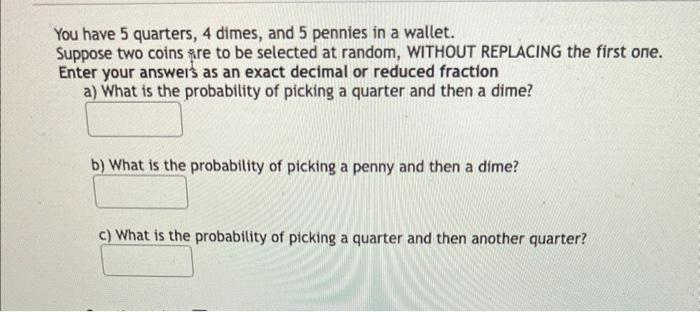Home / Expert Answers / Statistics and Probability / you-have-5-quarters-4-dimes-and-5-pennies-in-a-wallet-suppose-two-coins-are-to-be-selected-at-ra-pa166

# (Solved): You have 5 quarters, 4 dimes, and 5 pennies in a wallet. Suppose two coins are to be selected at ra ...You have 5 quarters, 4 dimes, and 5 pennies in a wallet. Suppose two coins are to be selected at random, WITHOUT REPLACING the first one. Enter your answeis as an exact decimal or reduced fraction a) What is the probability of picking a quarter and then a dime? b) What is the probability of picking a penny and then a dime? c) What is the probability of picking a quarter and then another quarter?

We have an Answer from Expert